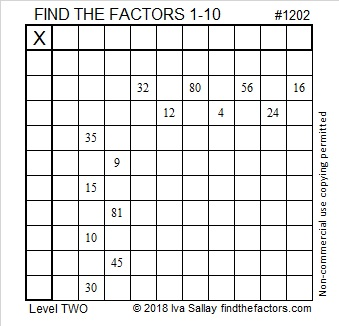1202 and Level 2

I am certain that you can fill in the numbers 1 to 10 one time in both the top row and the first column so that this puzzle can become a multiplication table. All you have to do is give it an honest try.Print the puzzles or type the solution in this excel file: 10-factors-1199-1210

Now I’ll write a few things about the number 1202:

• 1202 is a composite number.
• Prime factorization: 1202 = 2 × 601
• The exponents in the prime factorization are 1 and 1. Adding one to each and multiplying we get (1 + 1)(1 + 1) = 2 × 2 = 4. Therefore 1202 has exactly 4 factors.
• Factors of 1202: 1, 2, 601, 1202
• Factor pairs: 1202 = 1 × 1202 or 2 × 601
• 1202 has no square factors that allow its square root to be simplified. √1202 ≈ 34.6698729² + 19² = 1202

1202 is the hypotenuse of a Pythagorean triple:
480-1102-1202 calculated from 29² – 19², 2(29)(19), 29² + 19²

2(24² + 5²) = 2(601) = 1202 so that Pythagorean triple can also be calculated from
2(2)(24)(5), 2(24² – 5²), 2(24² + 5²)

Try out both ways to get the triple!

This site uses Akismet to reduce spam. Learn how your comment data is processed.